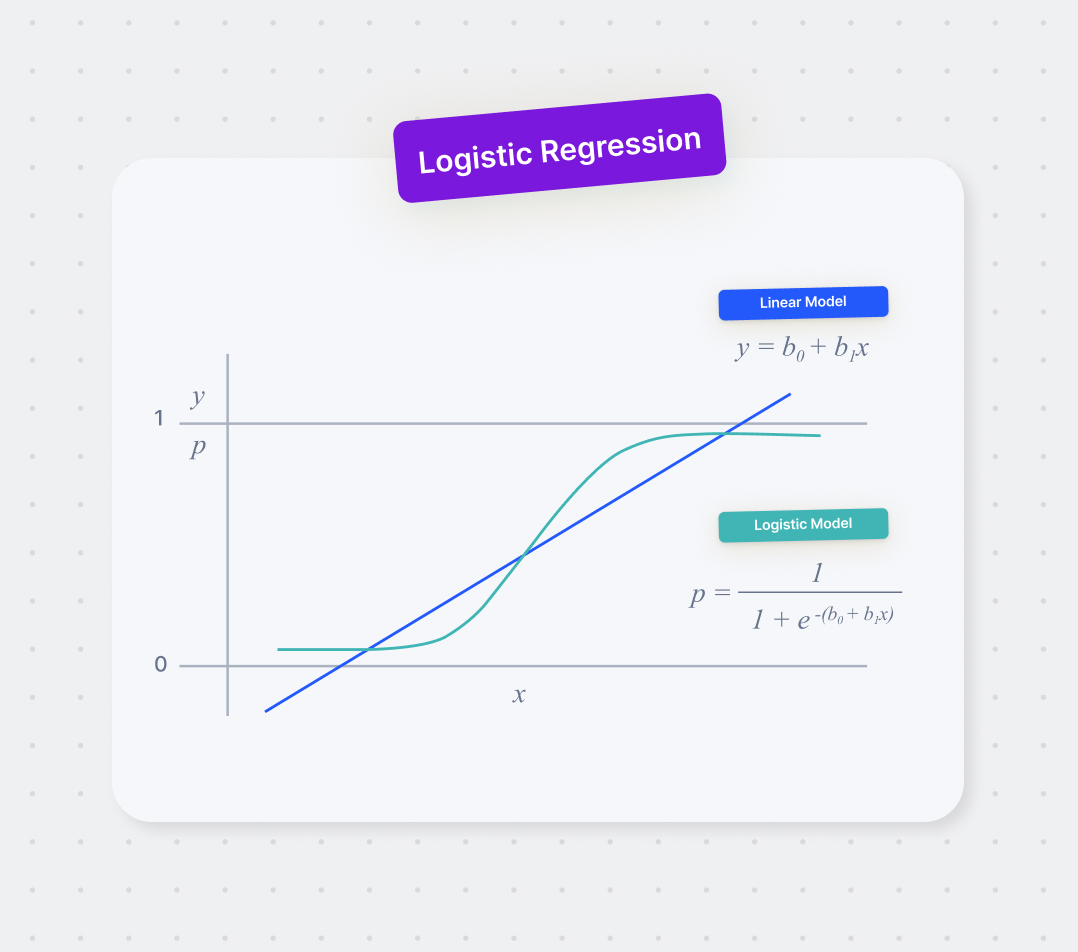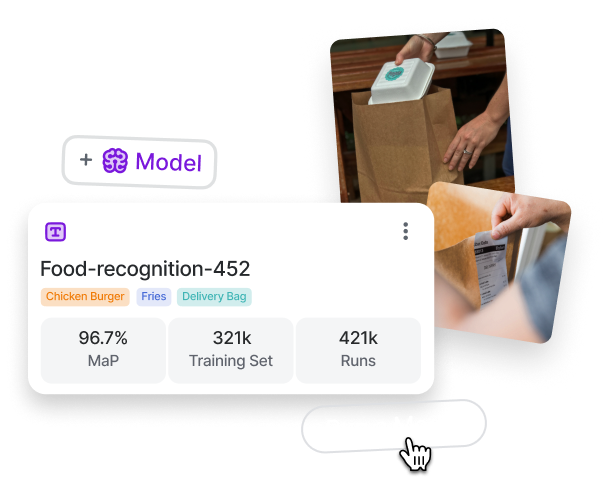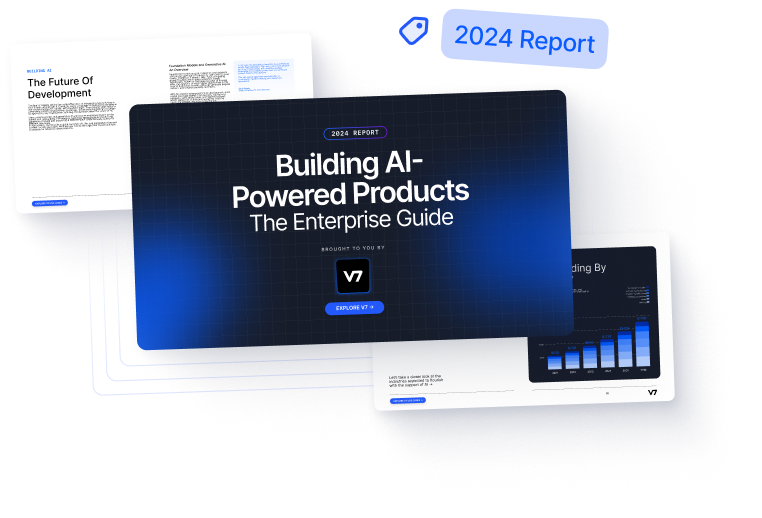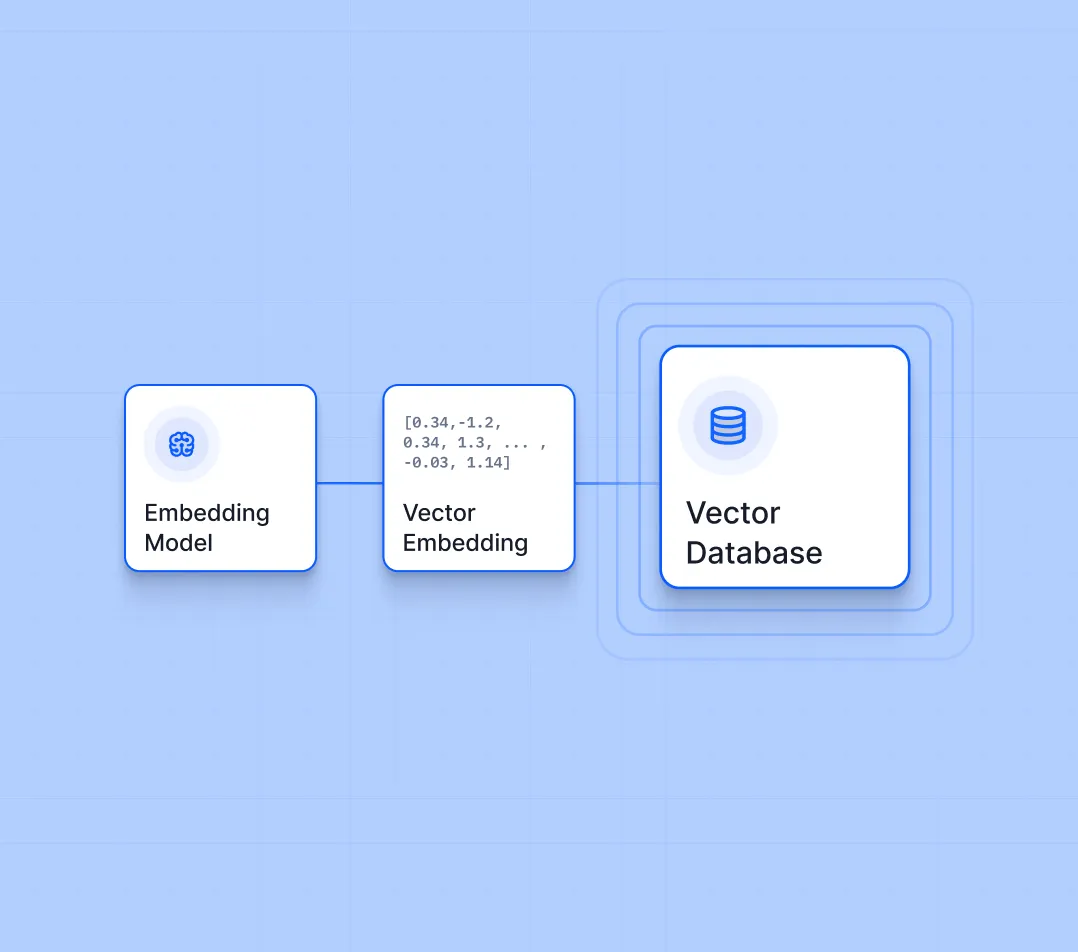# Logistic Regression: Definition, Use Cases, Implementation

Logistic regression is a popular classification algorithm, and the foundation for many advanced machine learning algorithms, Let's go through logistic regression basics, its real-life applications, and learn how to implement it.March 31, 2023Logistic regression is a popular classification algorithm, and the foundation for many advanced machine learning algorithms, including neural networks and support vector machines. It’s widely adapted in healthcare, marketing, finance, and more.

In logistic regression, the dependent variable is binary, and the independent variables can be continuous, discrete, or categorical. The algorithm aims to find the relationship between the input variables and the probability of the dependent variable being in one of the two categories.

In this article, we’ll give you a comprehensive overview of logistic regression, dive into the mathematical principles behind the algorithm, and provide practical examples of implementing it in PyTorch.

Here’s what we’ll cover:

Speed up labeling data 10x. Use V7 to develop AI faster.

Don't start empty-handed. Explore our repository of 500+ open datasets and test-drive V7's tools.

Ready to streamline AI product deployment right away? Check out:

## What is logistic regression?

Suppose you are an HR professional attempting to determine the status of an interview. Based on this status, you can check how it was conducted and decide whether to transfer the chosen applicants to the next round of interviews. The candidates must be categorized into "good" or "bad."

In this case, you can use logistic regression for outcome prediction since the predicted variable is dichotomous. The model considers several factors, often called independent variables $\left({x}_{i}\right)$>

Logistic regression is a classification model that uses several independent parameters to predict a binary-dependent outcome. It is a highly effective technique for identifying the relationship between data or cues or a particular occurrence.

Using a set of input variables, logistic regression aims to model the likelihood of a specific outcome. The output variable in logistic regression is binary—it may only assume one of two potential values (e.g., 0 for the event not to occur or 1 for the event to happen).

Any factors that impact the dependent variable are known as independent variables. Other than this, the input variables $\left({x}_{i}\right)$ may be categorical, continuous, or a combination of the two.

To convert the outcome into categorical value, we use the sigmoid function. The sigmoid function, which generates an S-shaped curve and delivers a probabilistic value ranging from 0 to 1, is used in machine learning to convert predictions to probabilities, as shown below. Although logistic regression is a linear technique, it alters the projections. The result is that, unlike linear regression, we can no longer comprehend the forecasts as a linear combination of the inputs.

The model can be written as follows:

$P(X)=eβ0+β1xi+βnxn1+eβ0+β1xi+βnxn$

This can also be rewritten as (note that f(x) and P(x) are being used interchangeably:

$P(x)=11+e-(X)$

where:

• e = the base of the natural logarithm
• x = linear combination of independent factors
• p(x) = probability that the event will occur (approximately 2.718).

Several explanatory factors frequently influence the quantity of the dependent variable's value. Logistic regression formulas assume the various independent variables are linearly related to the model. The output data variable can be computed by altering the sigmoid function as above and below:

The value of X Is calculated using the logistic regression equation:

$X=β0+β1x1+βnxn$

where:

• ${\beta }_{0}$ = level of intercept
• ${\beta }_{1}$ to ${\beta }_{n}$ = coefficients or weights
• ${x}_{1}$ to ${x}_{n}$ = independent variables

If x goes until infinity, predicted y becomes 1, and if it goes into negative infinity, y becomes 0. This is how the dependent variable's value is estimated through logistic regression.

Like linear regression, logit regression represents data with an equation showcasing a success ratio over failure. Log odds can also be calculated using the logit model.

Your odds are p/(1 - p) in terms of probability, and your odds equal log (p/(1 - p)). The logistic function can be represented as log odds, as seen below:

$log(p(X)1-P(X))=β0+βnxn$

where:

• $log\mathit{\left(}\frac{\mathit{p}\mathit{\left(}\mathit{X}\mathit{\right)}}{\mathit{1}\mathit{-}\mathit{P}\mathit{\left(}\mathit{X}\mathit{\right)}}\mathit{\right)}$ = logit function
• $\mathit{\left(}\frac{\mathit{p}\mathit{\left(}\mathit{X}\mathit{\right)}}{\mathit{1}\mathit{-}\mathit{P}\mathit{\left(}\mathit{X}\mathit{\right)}}\mathit{\right)}$ = odds

The ratio of the likelihood of success to the odds of failure is known as the odds. As a result, logistic regression converts a linear combination of inputs to log(odds), with an output of 1.

Although logistic regression is a linear technique, the logistic function alters the predictions, transforming them into a straight line using the odds. A threshold might be defined to determine the class.

Let’s go back to the interview status example. A sigmoid curve will be drawn based on the threshold values. These threshold values should be used when converting a probability value into a binary category. If the value is higher than the threshold, it's considered category 1; else it's considered category 2.

For instance, if p(x)= 0.98, which translates to a 98% chance for the event to occur, then it falls closer to category 1, and if p(x)= 0.28, it falls under category 2, which means there’s 28% chance for the event to occur. Machine learning thresholds must be adjusted because they depend on the situation, even if the default threshold is 0.5.

For simplicity, let’s have two classes, “good” or “bad,” with 1 = good and 0 = bad. The threshold value is assumed to be 0.5.

## Logistic regression assumptions

Assumptions are necessary for modeling a problem statement. They can be considered prerequisites for determining if you can appropriately make inferences from the analysis findings.

When picking a model, checking assumptions is crucial. Since every model has unique requirements, if the assumptions aren’t correct, the model may represent the data poorly and produce false predictions

Here’s the list of assumptions for logistic regression.

1. Linearity: A straight line should exist between the independent and dependent variables' log odds.
2. Independence: The observations should stand alone from one another. This implies that the response variable's value for a given observation shouldn't be tied to its value for any other observation.
3. No multicollinearity: There shouldn't be much correlation between the independent variables. It may be challenging to ascertain each predictor's impact on the response variable if there is a significant correlation between two or more predictors.
4. Large sample size: A substantial number of samples is needed to get accurate estimations of the coefficients.
5. No outliers: The findings of a logistic regression are presumed to be unaffected by extreme values or other outliers in the data. Outliers represent data points that significantly differentiate themselves from the remainder of the data and may profoundly affect the analysis findings. If outliers exist, they must be located and either eliminated or researched.

Many performance criteria can be used to assess the logistic regression model, including:

1. Accuracy: It gauges how many of the model's predictions were accurate.
2. Precision: It estimates the percentage of accurate positive predictions among all optimistic forecasts.
3. Recall: It measures the percentage of true positive predictions among all actual positive cases.
4. F1 score: A valuable metric for unbalanced datasets is the harmonic mean of precision and recall.

## Logistic regression vs. linear regression

Two standard statistical techniques used in various forms of data analysis are logistic regression and linear regression. Both approaches are applied to simulate the link between a dependent variable and one or more independent variables. However, logistic and linear regression differ fundamentally; each method is appropriate for specific issues.

1. Dependent variable: The dependent variable is binary in logistic regression. In linear regression,  the response variable is continuous (i.e., it takes only two values, 0 and 1). While logistic regression forecasts the likelihood of an event, linear regression forecasts a continuous outcome (i.e., the dependent variable being 1).
1. Assumptions: Linear regression counts on the residuals (i.e., the discrepancy between the predicted and actual values) being normally distributed and the linearity of the connection between the independent and dependent variables. Logistic regression assumes that the residuals remain distinct and equally distributed by a logistic distribution and that the log odds of the dependent variable are a linear mixture of the independent variables.
1. Output: Logistic regression results are probabilities between 0 and 1, while linear regression results are continuous numerical values. In logistic regression, the dependent variable is categorized as either 0 or 1 using a probability threshold (often 0.5).
1. Model selection: For a linear relationship between the independent factors and the dependent variable, linear regression is used. For a nonlinear relationship, logistic regression is used.
1. Interpretation: The coefficients show how the dependent variable changed for each unit change in the independent variable in linear regression.
1. Evaluation: Metrics such as mean squared error or R-squared is frequently used to assess the performance of a linear regression model, whereas accuracy, precision, and recall are commonly used to gauge the performance of a logistic regression model.
1. Applications: Logistic regression is frequently used to forecast binary outcomes, such as whether a consumer would buy a product, whereas linear regression is commonly used to indicate numerical results, such as sales or stock prices.
1. Data requirements: While logistic regression may handle non-normal data and non-linear correlations, linear regression implies that the data is usually distributed and has a linear connection between the dependent and independent variables.
2. Estimation technique: Whereas logistic regression is estimated using maximum likelihood estimation, linear regression is computed using the least-squares approach.

## Types of logistic regression

Let’s go through three types of logistic regression: binary, multinomial, and ordinal.

### Binary logistic regression

The most widely used type of logistic regression is binary logistic regression, applied when the dependent variable is binary or dichotomous. Using the values of one or more independent variables, binary logistic regression attempts to estimate the likelihood that the dependent variable will take on a specific value (such as 0 or 1) in the future. This particular form of logistic regression is helpful for forecasting outcomes, like whether a customer will purchase a product or not or whether a patient will benefit from a specific treatment.

While the binary regression model adjusts the result to the nearest values, the logistic function generates a range of values between 0 and 1. The logistic function typically provides a binary result by rounding values below 0.5 to 0 and values over 0.5 to 1.

### Multinomial logistic regression

Multinomial logistic regression is applied when the dependent variable includes more than two categories but no ordered subcategories. In other words, the categories have no inherent ordering; they are all mutually exclusive.

For example, a multinomial logistic regression model can be utilized to forecast the kind of fruit a consumer will purchase based on their demographic information. Apples, bananas, and oranges are conceivably produced. Another application of multinomial logistic regression models is image classification challenges, in which it is desired to categorize an image into one of the multiple groups.

Multinomial logistic regression works by mapping outcome values to different values between 0 and 1. Since the logistic function can return a range of continuous data, like 0.1, 0.11, 0.12, and so on, multinomial regression groups the output to the closest possible values.

### Ordinal logistic regression

Ordinal logistic regression is applied when the dependent variable includes more than two categories, and there is a natural ordering between the categories. For instance, research might be done to gauge a disease's severity from the patient's symptoms—with a range of potential outcomes, from minor to severe.

Ordinal logistic regression aims to simulate the relationship between the independent variables and the dependent variable's ordered categories. The change in the log chances of going from one category to the next higher category is represented by the coefficients in an ordinal logistic regression model.

Logistic regression is a flexible and effective method that may simulate the association between a binary or categorical dependent variable and one or more independent variables. It is possible to employ the numerous logistic regression models covered above to address various issues in various industries, such as marketing, healthcare, and picture categorization. Each of these models has its distinct advantages and applications.

Train ML models 10x faster

Turn labeled data into models. Develop production-ready AI in hours with just a few clicks.## Logistic regression implementation

Here’s the training code for logistic regression in PyTorch.

import torch
import torch.nn as nn
import torch.optim as optim
from torchvision.datasets import MNIST
from torchvision.transforms import ToTensor
from tqdm import tqdm

# Define the logistic regression model
class LogisticRegression(nn.Module):
def __init__(self):
super(LogisticRegression, self).__init__()
self.linear = nn.Linear(784, 10)  # 28*28=784

def forward(self, x):
x = x.view(-1, 784)  # flatten the image
out = self.linear(x)
return out

# Initialize the model, loss function, and optimizer
model = LogisticRegression()
criterion = nn.CrossEntropyLoss()
optimizer = optim.SGD(model.parameters(), lr=0.1)

# Train the model
for epoch in range(10):
running_loss = 0.0
outputs = model(images)
loss = criterion(outputs, labels)
loss.backward()
optimizer.step()
running_loss += loss.item()

# Test the model
correct = 0
total = 0
outputs = model(images)
_, predicted = torch.max(outputs.data, 1)
total += labels.size(0)
correct += (predicted == labels).sum().item()

print(f"Accuracy: {correct/total}")


This code is an implementation of logistic regression on the MNIST dataset using PyTorch. Here's a code breakdown:

1. Import the necessary libraries, including torch, and torch.nn, torch.optim, torch.utils.data, torchvision.datasets, and torchvision.transforms.
2. Define a class LogisticRegression that inherits from nn.Module. In the constructor, we define a linear layer with 784 input features (28*28) and 10 output features (since there are 10 classes in the MNIST dataset). In the forward method, we flatten the input tensor and pass it through the linear layer.
3. Load the MNIST data using torchvision.datasets.MNIST function and create data loaders for training and test sets using torch.utils.data.DataLoader.
4. Initialize the model, loss function, and optimizer. The model is an instance of LogisticRegression; the loss function is nn.CrossEntropyLoss and the optimizer are optim.SGD with a learning rate of 0.1.
5. Train the model for 10 epochs using a for loop. In each epoch, we iterate over the batches in the training data loader and perform the following steps:
b. Forward pass through the model using model(images)
c. Compute the loss using criterion(outputs, labels)
d. Backward pass through the model using loss.backward()
e. Update the model parameters using optimizer.step()
f. Track the running loss for the epoch using running_loss += loss.item()
6. Test the model on the test data using a for loop. We perform the following steps in each iteration:
a. Forward pass through the model using model(images)
b. Find the index with the highest score using torch.max(outputs.data, 1)
c. Compute the number of correct predictions using (predicted == labels).sum().item()
d. Track the total test samples using total += labels.size(0)
e. Track the number of correctly classified samples using correct += (predicted == labels).sum().item()
7. Print the accuracy of the model on the test set using print(f"Accuracy: {correct/total}").

## Logistic regression applications

Let's go through a few of the most popular applications of logistic regression across various industries.

### Optical character recognition

An optical character recognition (OCR) method, often called text recognition, may turn handwritten or printed characters into text that computers can understand. The output of optical character recognition is categorical, making it a classification challenge in machine learning (i.e., it belongs to a finite set of values). So, it warrants the use of logistic regression.

With logistic regression, we can train a binary classifier that can discriminate between distinct characteristics. The dependent variable in this instance is binary, denoting the presence or absence of a character. The features retrieved from the input image are the independent variables.

The initial step in OCR is to take the input image's features and extract them. Features used to characterize an image include lines, curves, and edges. Following their extraction, parts can be fed into a machine-learning model such as logistic regression. The weights of the independent factors that predict the likelihood of the observed data are estimated by logistic regression. These coefficients are applied to new image forecasts.

### Fraud detection

Fraud detection is about detecting scams and preventing fraudsters from acquiring money or property by deception. Fraud poses a significant financial liability that must be discovered and mitigated immediately.

Many organizations struggle with fraud detection, particularly finance, insurance, and e-commerce. Using machine learning methods such as logistic regression is one strategy for fraud detection. It can be used to train a binary classifier that can discriminate between fraudulent and legitimate transactions.

The dependent variable, in this instance, is binary and represents if the payment is fraudulent or not. The elements that characterize a transaction, including its value, place, time, and user details, are its independent variables. The effectiveness of fraud detection can be increased by combining logistic regression with other machine learning methods like anomaly detection and decision trees.

##### 💡 Read more:9 Innovative Use Cases of AI in Finance

Disease spread prediction can be approached as a binary classification problem, where the target variable is whether an individual will contract the disease.

Data including the number of affected people, the population's age and health, the environment, and the accessibility of medical resources, can affect how quickly diseases spread. The link between these variables and the risk of disease transmission can be modeled using logistic regression.

A dataset of historical disease spread data can be used to predict the spread of illnesses using logistic regression. The dataset should contain details regarding the number of affected people, the time frame, and the place. To increase the accuracy of disease spread prediction, we can combine logistic regression with other machine learning methods, such as time series analysis and clustering. The temporal patterns of disease propagation can be modeled using time series analysis. Clustering can be used to pinpoint the areas and populations that are most impacted.

### Illness mortality prediction

Logistic regression is widely used for mortality prediction to calculate the likelihood of a person dying with a specific illness.

Data attributes like demographics, health information, and clinical information like age, gender, conditions, and clinical indicators, including hypertension, heart rate, and lab test results, can be used to train the logistic regression model. This can assist medical professionals in making wise choices regarding patient care and enhance patient outcomes.

### Churn prediction

Churn prediction identifies customers likely to stop using a product or service. Logistic regression is a commonly used technique to model churn prediction.

In logistic regression, the dependent variable is a binary variable that indicates whether a customer will churn. The independent variables are the customer's demographic information, usage patterns, and other factors that may influence their decision to leave.

The logistic regression model estimates each customer's churn probability, based on the independent variables. The model then predicts a customer's churn based on a threshold probability.

The logistic regression model can identify customers at high risk of churning, enabling businesses to take proactive measures to retain them. This can include targeted marketing campaigns, personalized offers, and customer support interventions.

## Key takeaways

Logistic regression is a popular machine learning algorithm used for binary classification problems, where the goal is to predict the probability of an event occurring. Here are some key takeaways:

• Logistic regression is a supervised learning algorithm for binary classification tasks.
• It works by modeling the relationship between the independent variables (predictors) and the dependent variable (target) using a sigmoid function, which outputs a probability between 0 and 1.
• The algorithm uses optimization techniques to find the best coefficients for the independent variables that maximize the likelihood of the observed data.
• Logistic regression is a linear model, assuming a linear relationship between the predictors and the target variable.
• It is a parametric model, which assumes a specific distribution for the error terms in the model.

References

1. Alenzi, H. Z., & Aljehane, N. O. (2020). Fraud detection in credit cards using logistic regression. International Journal of Advanced Computer Science and Applications, 11(12).
2. Hazra, T. K., Sarkar, R., & Kumar, A. (2016). Handwritten English character recognition using logistic regression and neural network. Int. J. Sci. Res, 5, 750-754.
3. LaValley, M. P. (2008). Logistic regression. Circulation, 117(18), 2395-2399.
4. Shalizi, C. (n.d.). Logistic Regression. Department of Statistics. Retrieved February 16, 2023, from https://www.stat.cmu.edu/~cshalizi/uADA/12/lectures/ch12.pdf
5. Zhang, Z. (2016). Model building strategy for logistic regression: purposeful selection. Annals of translational medicine, 4(6).Deval is a senior software engineer at Eagle Eye Networks and a computer vision enthusiast. He writes about complex topics related to machine learning and deep learning.

“Collecting user feedback and using human-in-the-loop methods for quality control are crucial for improving Al models over time and ensuring their reliability and safety. Capturing data on the inputs, outputs, user actions, and corrections can help filter and refine the dataset for fine-tuning and developing secure ML solutions.”Name
Company
GUIDE
Building AI-Powered Products: The Enterprise Guide

Building AI products? This guide breaks down the A to Z of delivering an AI success story.

Oops! Something went wrong while submitting the form.## Related articlesVector Databases: Intro, Use Cases, Top 5 Vector DBs
Deval Shah15